# Fundamental Theorem of Calculus

The fundamental theorem of calculus specifies the relationship between the two central operations of calculus: differentiation and integration.

The first part of the theorem, sometimes called the first fundamental theorem of calculus, shows that an indefinite integration can be reversed by a differentiation.

More exactly, the theorem deals with definite integration with variable upper limit and arbitrarily selected lower limit. This particular kind of definite integration allows us to compute one of the infinitely many antiderivatives of a function (except for those which do not have a zero). Hence, it is almost equivalent to indefinite integration, defined by most authors as an operation which yields any one of the possible antiderivatives of a function, including those without a zero. Integration can be reversed by a differentiation. The first part is also important because it guarantees the existence of antiderivatives for continuous functions.

The second part, sometimes called the second fundamental theorem of calculus, allows one to compute the definite integral of a function by using any one of its infinitely many antiderivatives. This part of the theorem has invaluable practical applications, because it markedly simplifies the computation of definite integrals.

The first published statement and proof of a restricted version of the fundamental theorem was by James Gregory. Isaac Barrow proved the first completely general version of the theorem, while Barrow's student, Isaac Newton, completed the development of the surrounding mathematical theory. Gottfried Leibniz systematized the knowledge into a calculus for infinitesimal quantities.

## Physical intuition

Intuitively, the theorem simply states that the sum of infinitesimal changes in a quantity over time (or over some other quantity) adds up to the net change in the quantity.

In the case of a particle traveling in a straight line, its position, x, is given by x(t) where t is time and x(t) means that x is a function of t. The derivative of this function is equal to the infinitesimal change in quantity, dx, per infinitesimal change in time, dt (of course, the derivative itself is dependent on time). This change in displacement per change in time is the velocity v of the particle. In Leibniz notation: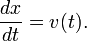Rearranging this equation, it follows that: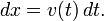By the logic above, a change in x (or Δx) is the sum of the infinitesimal changes dx. It is also equal to the sum of the infinitesimal products of the derivative and time. This infinite summation is integration; hence, the integration operation allows the recovery of the original function from its derivative. It can be concluded that this operation works in reverse; the result of the integral can be differentiated to recover the original function.

## Formal statements

There are two parts to the Fundamental Theorem of Calculus. Loosely put, the first part deals with the derivative of an antiderivative, while the second part deals with the relationship between antiderivatives and definite integrals.

### First part

This part is sometimes referred to as the First Fundamental Theorem of Calculus.

Let ƒ be a continuous real-valued function defined on a closed interval [a, b]. Let F be the function defined, for all x in [a, b], by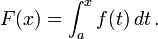Then, F is continuous on [a, b], differentiable on the open interval (ab), and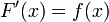for all x in (a, b).

### Corollary

The fundamental theorem is often employed to compute the definite integral of a function ƒ for which an antiderivative g is known. Specifically, if ƒ is a real-valued continuous function on [ab], and g is an antiderivative of ƒ in [ab], then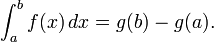The corollary assumes continuity on the whole interval. This result is strengthened slightly in the following theorem.

### Second part

This part is sometimes referred to as the Second Fundamental Theorem of Calculus or the Newton-Leibniz Axiom.

Let ƒ be a real-valued function defined on a closed interval [a, b] that admits an antiderivative g on [ab]. That is, ƒ and g are functions such that for all x in [ab],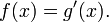If ƒ is integrable on [ab] then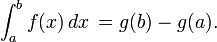Notice that the Second part is somewhat stronger than the Corollary because it does not assume that ƒ is continuous.

Note that when an antiderivative g exists, then there are infinitely many antiderivatives for ƒ, obtained by adding to g an arbitrary constant. Also, by the first part of the theorem, antiderivatives of ƒ always exist when ƒ is continuous.

## Proof

The proof has been omitted from Skulepedia because only EngScis need to know that, and they aren't real people.